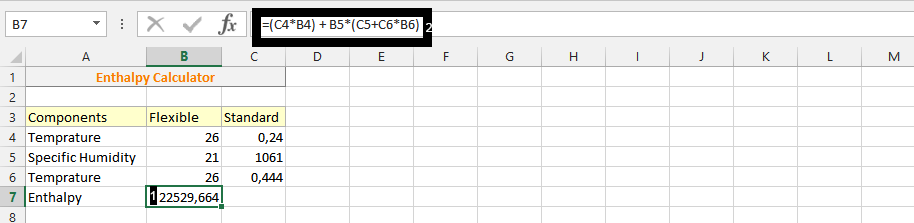#### How to calculate enthalpy change?

The calculation of enthalpy is possibly done in a very simple way, and it is because it makes it possible to find the right answer.

## What is enthalpy?

Enthalpy is a thermodynamic property that is defined as the sum of the internal energy of a system and the product of its pressure and volume. It is often used to measure the heat content of a system.

## How to calculate enthalpy in Excel?

The data should include the temperature, pressure, and specific heat capacity of the system.

This is just an example data set which contains temperature and humidity values.

Click on an empty cell, and enter the formula =(C4*B4) + B5*(C5+C6*B6).

It is based on the following equation:

`Enthalpy = Specific heat capacity * Mass * Temperature change`Enthalpy change has been calculated.

You can also use the ENTROPY function in Excel to calculate enthalpy. The ENTROPY function takes into account the pressure and volume of the system as well. The formula for the ENTROPY function is:

`ENTROPY = Specific heat capacity * Mass * Temperature change * (1 + Pressure / Volume)`